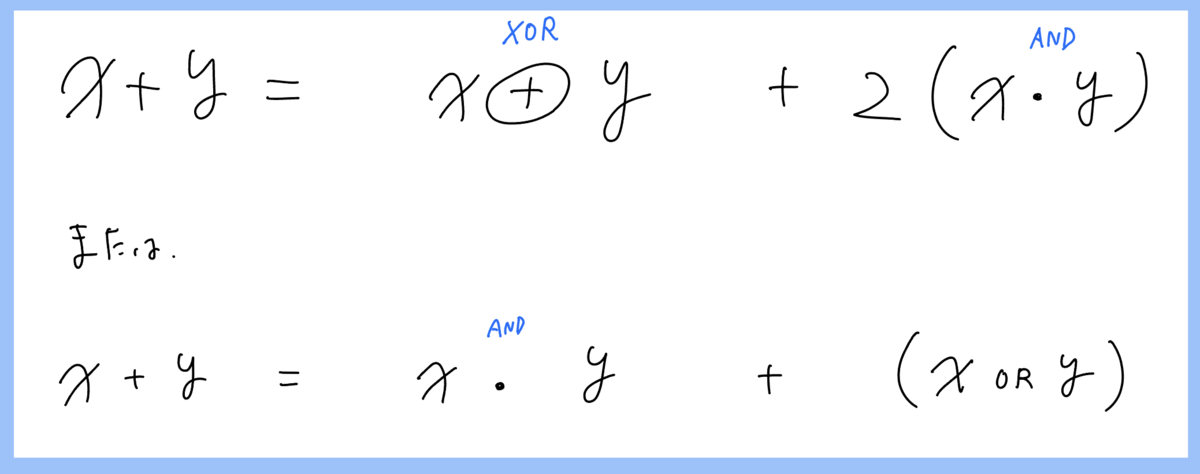# ダイクストラ 復元 注意点 prev

```struct Node{
ll distance;
ll index;
ll prev=-1;
};

// ダイクストラアルゴリズム内部
{ // 省略
while(!que.empty()){
// 1番distanceが小さいノード
Node n = que.top();que.pop();

if(done[n.index])continue;

// 確定
done[n.index] = true;
d[n.index] = n.distance;
prev[n.index] = n.prev; // ここでprevを上書きすべき★1

auto edge_list = graph[n.index];
for(auto edge : edge_list){
// 短くなるノードがあれば
if(!done[edge.to] && n.distance + edge.cost < d[edge.to]){
ll shorter_distance = n.distance + edge.cost;
que.push(Node(shorter_distance, edge.to, n.index));
// prev[edge.to] = n.index; // ここに書いてもバグります★2
}
}
}
}
```
• 具体的には★1の箇所でprevに入力しなければならなかった
• 最初、★2のところでprevを書き換えたがそれはミス。edge.toの距離が3のものをpush, edge.toの距離が4のものをpush, とした場合、距離4の方でprevが上書きされてしまうため

# (自分用メモ) 論理式の式変形 (和をXOR, ANDで書き直す)# C - Remembering the Days next_permutation解法

```int main(){
cin.tie(0);
ios::sync_with_stdio(false);

// input
ll N,M;
cin>>N>>M;

VV A(N, VI(N, -1));

rep(_,M){
ll a,b,c;cin>>a>>b>>c;
a--;b--;
A[a][b]=c;
A[b][a]=c;
}

ll ma=0;
VI I;
rep(i,N)I.push_back(i);

do{
// Iの順に行く
ll sum=0;
rep(i,N-1){
ll from = I[i];
ll to = I[i+1];
if(A[from][to]==-1){
sum=-inf;
}else{
sum += A[from][to];
}
chmax(ma,sum); // 毎回取る
}
}while(next_permutation(ALL(I)));

p(ma);

return 0;
}
```

# 最短ハミルトンパス

• 頂点数は17以下を想定
• 隣接行列を与えるとパスを返す関数
```// ハミルトンパスが存在するなら返す
// （無いなら空の配列を返す）
// 入力 G : 隣接行列 (adjacency matrix)
// 参考にした : by tatyam
//   atcoder.jp/contests/abc190/submissions/19761405
VI Get_Hamiltonian_Path(VV G){
ll N = G.size();
assert(N<30);
// dp[flag][last]
// flag : visit flags
// last : last position
VV dp(1<<N, VI(N,inf));
rep(i,N){
dp[1<<i][i]=0; // 始点のみ訪れた場合
}
FOR(after,1,1<<N){
rep(i,N) if(after & 1<<i){ // iを削る
ll before = after ^ 1<<i;
rep(j,N) if(before & 1<<j){
// beforeの状態でラストがjの点から、iへの遷移
chmin(dp[after][i], dp[before][j] + G[j][i]);
}
}
}
ll min_len = inf;
ll min_last = -1;
rep(i,N){
ll all = (1<<N)-1;
if(dp[all][i] < min_len){
min_len = dp[all][i];
min_last = i;
}
}
if(min_len==inf){
VI path; return path;
}
// 復元
VI path = {min_last};
ll visit = (1<<N)-1;
ll last = min_last;
ll len = min_len;
while(len){
rep(i,N) if(visit & 1<<i){
// iからlastに来たかどうか
if(i==last)continue;
ll before = visit ^ (1<<last);
if(dp[before][i] + G[i][last] == dp[visit][last]){
// iから来たんだね
visit = before;
len -= G[i][last];
last = i;
path.push_back(i);
break;
}
}
}
reverse(ALL(path));
return path;
}
```

# abc309_c "C - Medicine" 二分探索解法

```int main(){
cin.tie(0);
ios::sync_with_stdio(false);

// input
ll N,K;
cin>>N>>K;

VI A(N);
VI B(N);
rep(i,N){
cin >> A[i] >> B[i];
}

// 1日目からK錠以下かどうか
if(SUM(B)<=K){
p(1);
return 0;
}

ll left=1; // K錠より大きい
ll right=inf; // K錠以下

while(right-left>1){
// centerの日
ll center = (left+right)/2;

// centerの日に飲むタブレットの合計
ll tablet=0;

rep(i,N){
if(A[i]>=center){
tablet += B[i];
}
}

if(tablet<=K){
right = center;
}else{
left = center;
}
}
p(right);

return 0;
}
```